Courses

# Legendre Special Function (Part - 1)- Mathematical Methods of Physics, UGC - NET Physics Physics Notes | EduRev

## Physics for IIT JAM, UGC - NET, CSIR NET

Created by: Akhilesh Thakur

## Physics : Legendre Special Function (Part - 1)- Mathematical Methods of Physics, UGC - NET Physics Physics Notes | EduRev

The document Legendre Special Function (Part - 1)- Mathematical Methods of Physics, UGC - NET Physics Physics Notes | EduRev is a part of the Physics Course Physics for IIT JAM, UGC - NET, CSIR NET.
All you need of Physics at this link: Physics

GENERATING FUNCTION

Legendre polynomials appear in many different mathematical and physical situations. (1) They may originate as solutions of the Legendre ODE which we have already encountered in the separation of variables for Laplace’s equation, Helmholtz’s equation, and similar ODEs in spherical polar coordinates. (2) They enter as a consequence of a Rodrigues’ formula. (3) They arise as a consequence of demanding a complete, orthogonal set of functions over the interval [−1, 1] (Gram–Schmidt orthogonalization . (4) In quantum mechanics they (really the spherical harmonics, represent angular momentum eigenfunctions. (5) They are generated by a generating function. We introduce Legendre polynomials here by way of a generating function.

Physical Basis — Electrostatics

As with Bessel functions, it is convenient to introduce the Legendre polynomials by means of a generating function, which here appears in a physical context. Consider an electric charge q placed on the z-axis at z = a . As shown in Fig. 12.1, the electrostatic potential of charge q is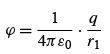(SI units).                        (12.1)

We want to express the electrostatic potential in terms of the spherical polar coordinates r and θ (the coordinate ϕ is absent because of symmetry about the z-axis). Using the law of cosines in Fig. 12.1, we obtain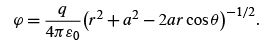(12.2)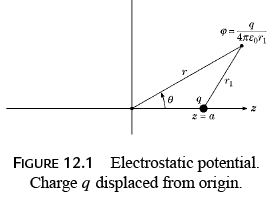Legendre Polynomials

Consider the case of r> a or, more precisely, r 2 > |a 2 − 2ar cos θ |. The radical in Eq. (12.2) may be expanded in a binomial series and then rearranged in powers of (a / r ).
The Legendre polynomial Pn (cos θ) (see Fig. 12.2) is deﬁned as the coefﬁcient of the nth power in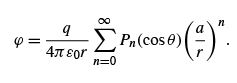(12.3)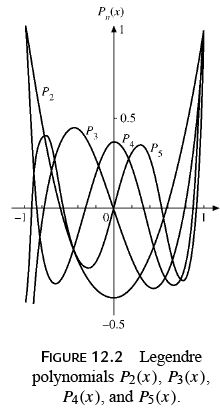Dropping the factor q/4πε0 r and using x and t instead of cos θ and a/ r , respectively, we have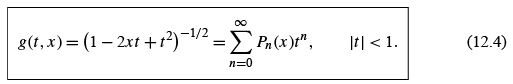Equation (12.4) is our generating function formula. In the next section it is shown that |Pn (cos θ)|≤ 1, which means that the series expansion (Eq. (12.4)) is convergent for |t | < 1.1 Indeed, the series is convergent for |t |= 1 except for |x |= 1.
In physical applications Eq. (12.4) often appears in the vector form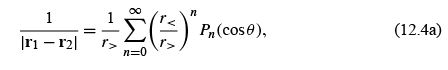where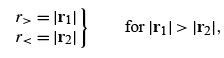(12.4b)

and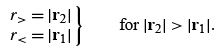(12.4c)

Using the binomial theorem (Section 5.6) and Exercise 8.1.15, we expand the generating function as (compare Eq. (12.33))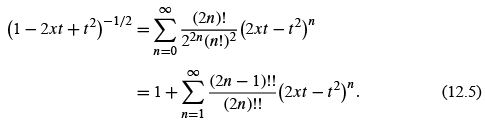For the ﬁrst few Legendre polynomials, say, P0 ,P1 , and P2 , we need the coefﬁcients of t0 , t1 , and t2 . These powers of t appear only in the terms n = 0, 1, and 2, and hence we may limit our attention to the ﬁrst three terms of the inﬁnite series: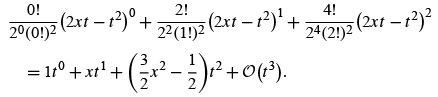Then, from Eq. (12.4) (and uniqueness of power series),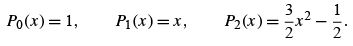We repeat this limited development in a vector framework later in this section.

In employing a general treatment, we ﬁnd that the binomial expansion of the (2xt − t 2)n factor yields the double seriesFrom Eq. (5.64)  (rearranging the order of summation), Eq. (12.6) becomes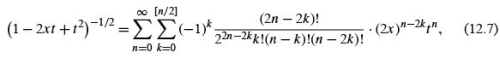with the t n independent of the index k . Now, equating our two power series (Eqs. (12.4) and (12.7)) term by term, we have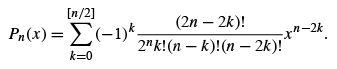(12.8)

Hence, for n even, Pn has only even powers of x and even parity (see Eq. (12.37)), and odd powers and odd parity for odd n.

Linear Electric Multipoles

Returning to the electric charge on the z-axis, we demonstrate the usefulness and power of the generating function by adding a charge −q at z =−a , as shown in Fig. 12.3. The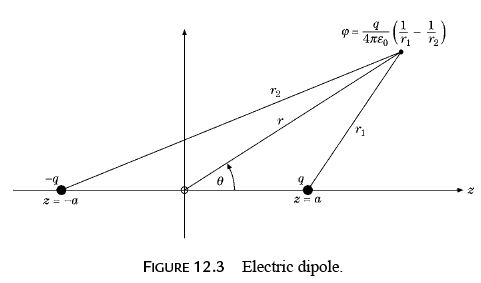potential becomes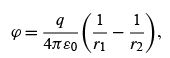(12.9)
and by using the law of cosines, we have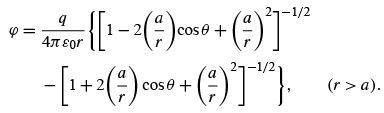Clearly, the second radical is like the ﬁrst, except that a has been replaced by −a . Then, using Eq. (12.4), we obtain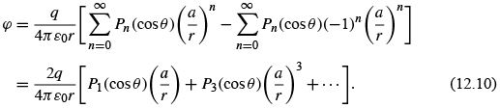The ﬁrst term (and dominant term for r ≫ a )is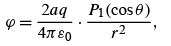(12.11)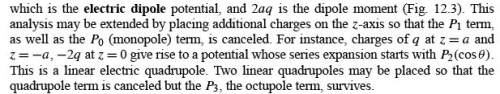Vector Expansion

We consider the electrostatic potential produced by a distributed charge ρ(r2 ):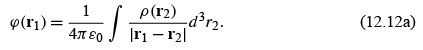This expression has already appeared in Sections 1.16 and 9.7. Taking the denominator of the integrand, using ﬁrst the law of cosines and then a binomial expansion, yields (see Fig. 1.42)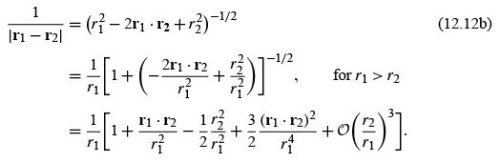(For r1 = 1,r= t , and r1 · r2 = xt , Eq. (12.12b) reduces to the generating function, Eq. (12.4).) The ﬁrst term in the square bracket, 1, yields a potential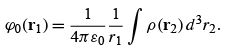(12.12c)

The integral is just the total charge. This part of the total potential is an electric monopole.
The second term yields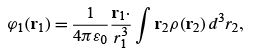(12.12d)

where the integral is the dipole moment whose charge density ρ(r2 ) is weighted by a moment arm r2 . We have an electric dipole potential. For atomic or nuclear states of deﬁnite parity, ρ(r2 ) is an even function and the dipole integral is identically zero.
The last two terms, both of order (r2 /r1), may be handled by using Cartesian coordinates: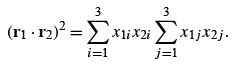Rearranging variables to take the xcomponents outside the integral yields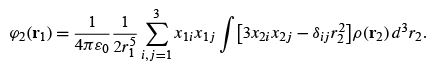(12.12e)

This is the electric quadrupole term. We note that the square bracket in the integrand forms a symmetric, zero-trace tensor.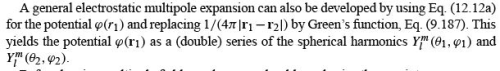Before leaving multipole ﬁelds, perhaps we should emphasize three points.

• First, an electric (or magnetic) multipole is isolated and well deﬁned only if all lowerorder multipoles vanish. For instance, the potential of one charge q at z = a was expanded in a series of Legendre polynomials. Although we refer to the P1 (cos θ) term in this expansion as a dipole term, it should be remembered that this term exists only because of our choice of coordinates. We also have a monopole, P0 (cos θ).
• Second, in physical systems we do not encounter pure multipoles. As an example, the potential of the ﬁnite dipole ( q at z = a, −q at z =−a ) contained a P3 (cos θ) term. These higher-order terms may be eliminated by shrinking the multipole to a point multipole, in this case keeping the product qa constant (a → 0,q →∞) to maintain the same dipole moment.
•  Third, the multipole theory is not restricted to electrical phenomena. Planetary conﬁgurations are described in terms of mass multipoles, Gravitational radiation depends on the time behavior of mass quadrupoles. (The gravitational radiation ﬁeld is a tensor ﬁeld. The radiation quanta, gravitons, carry two units of angular momentum.)

It might also be noted that a multipole expansion is actually a decomposition into the irreducible representations of the rotation group.

Extension to Ultraspherical Polynomials

The generating function used here, g(t , x ), is actually a special case of a more general generating function,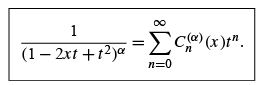(12.13)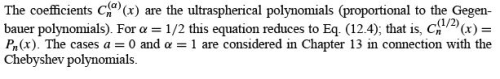RECURRENCE RELATIONS AND SPECIAL PROPERTIES

Recurrence Relations

The Legendre polynomial generating function provides a convenient way of deriving the recurrence relations4 and some special properties. If our generating function (Eq. (12.4)) is differentiated with respect to t , we obtain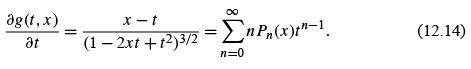By substituting Eq. (12.4) into this and rearranging terms, we have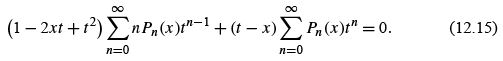The left-hand side is a power series in t . Since this power series vanishes for all values of t , the coefﬁcient of each power of t is equal to zero; that is, our power series is unique (Section 5.7). These coefﬁcients are found by separating the individual summations and using distinctive summation indices: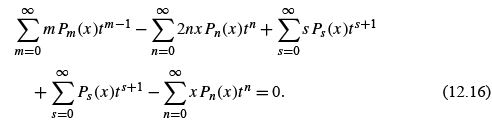Now, letting m = n + 1,s = n − 1, we ﬁnd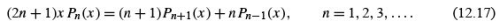.This is another three-term recurrence relation, similar to (but not identical with) the recurrence relation for Bessel functions. With this recurrence relation we may easily construct the higher Legendre polynomials. If we take n = 1 and insert the easily found values of P0 (x ) and P1 (x ) (Exercise 12.1.7 or Eq. (12.8)), we obtain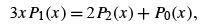(12.18)

or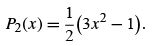(12.19)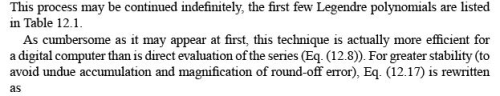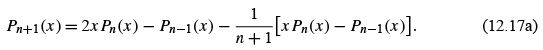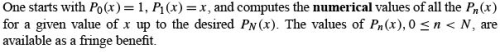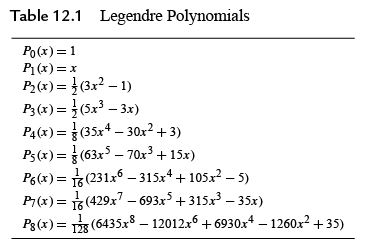Differential Equations

More information about the behavior of the Legendre polynomials can be obtained if we now differentiate Eq. (12.4) with respect to x . This gives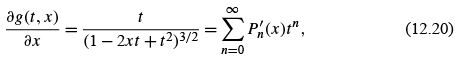or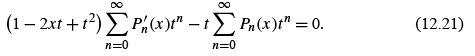As before, the coefﬁcient of each power of t is set equal to zero and we obtain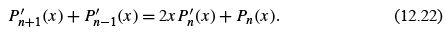A more useful relation may be found by differentiating Eq. (12.17) with respect to x and multiplying by 2. To this we add (2n + 1) times Eq. (12.22), canceling the Pn′ term. The result isFrom Eqs. (12.22) and (12.23) numerous additional equations may be developed,5 including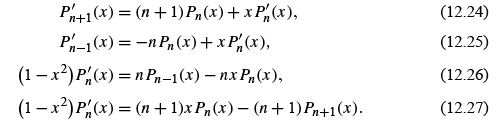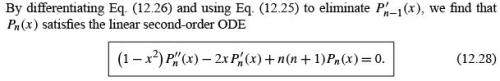The previous equations, Eqs. (12.22) to (12.27), are all ﬁrst-order ODEs, but with polynomials of two different indices. The price for having all indices alike is a second-order differential equation. Equation (12.28) is Legendre’s ODE. We now see that the polynomials Pn (x ) generated by the power series for (1 − 2xt + t 2 )−1/2 satisfy Legendre’s equation, which, of course, is why they are called Legendre polynomials.
In Eq. (12.28) differentiation is with respect to x(x = cos θ). Frequently, we encounter Legendre’s equation expressed in terms of differentiation with respect to θ :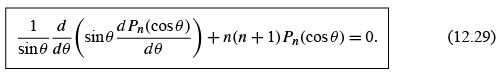Special Values

If we set x = 1, Eq. (12.4) becomes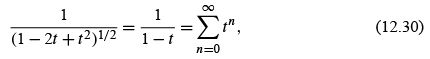using a binomial expansion or the geometric series, Example 5.1.1. But Eq. (12.4) for x = 1 deﬁnes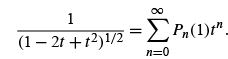Comparing the two series expansions (uniqueness of power series, Section 5.7), we have

Pn (1) = 1.                           (12.31)

If we let x =−1 in Eq. (12.4) and use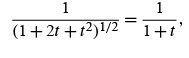this shows that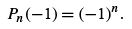(12.32)

For obtaining these results, we ﬁnd that the generating function is more convenient than the explicit series form, Eq. (12.8).
If we take x = 0 in Eq. (12.4), using the binomial expansion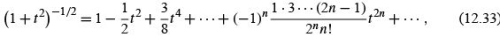we have6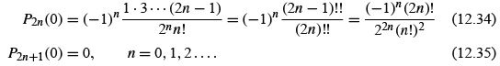These results also follow from Eq. (12.8) by inspection.

Parity

Some of these results are special cases of the parity property of the Legendre polynomials.

We refer once more to Eqs. (12.4) and (12.8). If we replace x by −x and t by −t ,the generating function is unchanged. Hence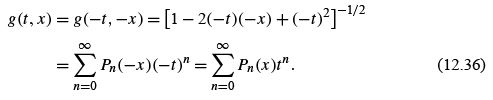Comparing these two series, we have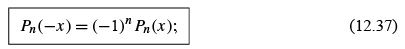that is, the polynomial functions are odd or even (with respect to x = 0,θ = π/2) according to whether the index n is odd or even. This is the parity,7 or reﬂection, property that plays such an important role in quantum mechanics. For central forces the index n is a measure of the orbital angular momentum, thus linking parity and orbital angular momentum.
This parity property is conﬁrmed by the series solution and for the special values tabulated in Table 12.1. It might also be noted that Eq. (12.37) may be predicted by inspection of Eq. (12.17), the recurrence relation. Speciﬁcally, if Pn−1 (x ) and xPn (x ) are even, then Pn+1 (x ) must be even.

Upper and Lower Bounds for Pn(cos θ)

Finally, in addition to these results, our generating function enables us to set an upper limit on |Pn (cos θ)|.Wehave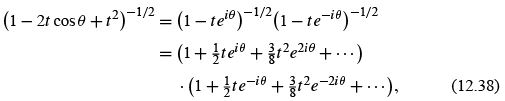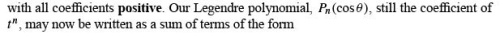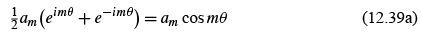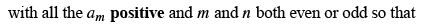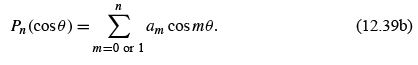This series, Eq. (12.39b), is clearly a maximum when θ = 0 and cos mθ = 1. But for x = cos θ = 1, Eq. (12.31) shows that P(1) = 1. Therefore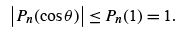(12.39c)

A fringe beneﬁt of Eq. (12.39b) is that it shows that our Legendre polynomial is a linear combination of cos mθ . This means that the Legendre polynomials form a complete set for any functions that may be expanded by a Fourier cosine series (Section 14.1) over the interval [0,π ].

• In this section various useful properties of the Legendre polynomials are derived from the generating function, Eq. (12.4).
• The explicit series representation, Eq. (12.8), offers an alternate and sometimes superior approach.
Offer running on EduRev: Apply code STAYHOME200 to get INR 200 off on our premium plan EduRev Infinity!

159 docs

,

,

,

,

,

,

,

,

,

,

,

,

,

,

,

,

,

,

,

,

,

,

,

,

;